# Software that writes software: the state of program synthesis

Writing correct software is surprisingly hard.

This collection of prominent software post-mortems by Dan Luu is a humbling reminder of this harrowing truth. It features Knight Capital's $459 million loss in 45 minutes due to conflicting deployed software versions, and a Linux kernel bug related to leap-second handling taking down much of the Internet - Amazon Web Services, Reddit, and Mozilla included. This motivates a question - is it possible to write software that writes software? By one definition, we already have; compilers, which programmers use every day, are ubiquitous. While it is theoretically possible to write optimized x86 assembly code, the magnificent GNU Compiler Collection (gcc) allows us to program in C, a higher level programming language. Still, this is an unsatisfying example. While compilers let us program with greater levels of abstraction, at the end of the day, humans still need to write code. A better question to ask might be: is it possible to generate useful programs directly from a list of requirements, without human intervention? Recent research efforts have contended with this problem, with early but promising results. Perhaps the most compelling motivation to consider this problem is program complexity. Software that writes software might produce more sophisticated, flexible systems than humans are capable of designing. A recent research paper by Google Brain applied this idea to designing neural network architectures; the algorithmically designed tf.contrib.rnn.NASCell was even merged into TensorFlow.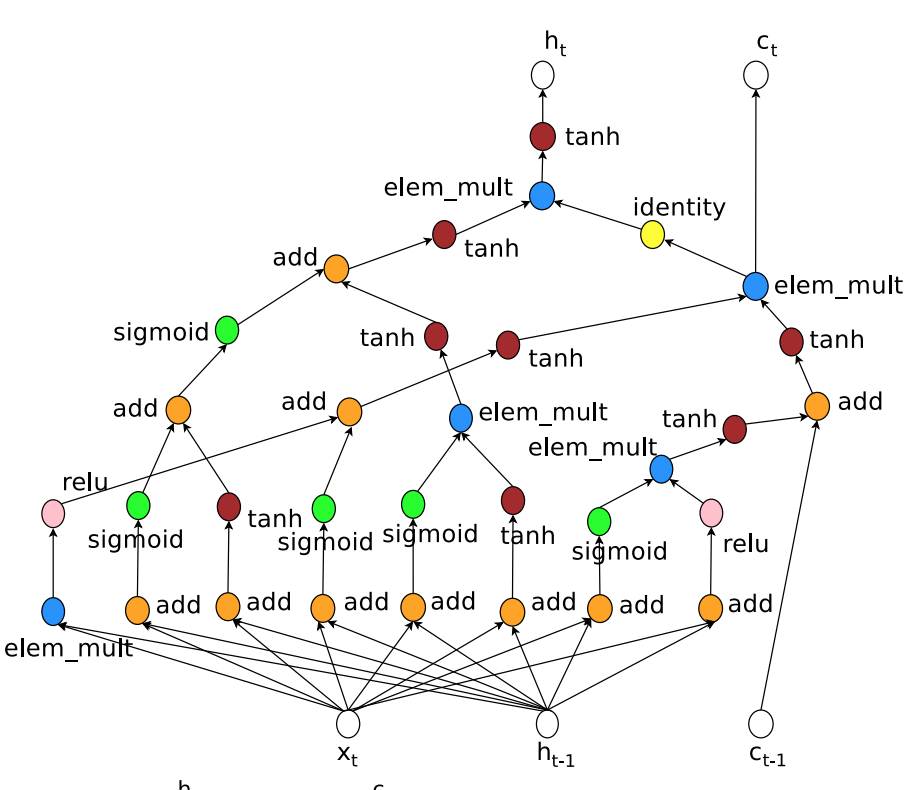As Smolensky asserted in 1987, there have been two broad paradigms in AI: symbolic and connectionist. Symbolic systems operate on entities (“symbols”) that are representations of data. The classic example is expert systems, in which human experts hard code knowledge. For instance, a stock trader might encode the rule “if GOOGL stock falls below$900, sell 1000 shares.”

Connectionist systems can be described as “large networks of extremely simple processors, massively interconnected and running in parallel” (Smolensky). Neural networks, of course, fall into this camp. These systems tend to apply statistical inference techniques, and operate on numeric features. These statistical systems tend to be less interpretable, but often perform better, given more training data.

Programs that write programs are interesting because it fuses the symbolic and the connectionist in a unique way.

They are symbolic in that programming languages provide a syntactic, human interpretable language for computation. They are connectionist in that the final output code is often computed with the assistance of neural networks, gradient descent, and other statistical tools.

It seems that the field of program induction has been less noticed than more conventional supervised approaches in deep learning. This might be because the learned programs are relatively simple. For example, DeepCoder (Balog et al. 2017) is able to construct programs of only up to 5-10 lines. While the practical applications of these programs are limited, being able to synthesize them at all over a large search space is a significant research milestone.

## Machine learning as searching the program space

Supervised machine learning can be thought of as “searching a program space.” In particular, we have some target behavior, for instance “identify vehicles in images,” that we do not know how to encode procedurally. We encode priors about this particular in a model choice; we might opt to choose convolutional neural networks to learn features on image data. And the training algorithm searches the program space for the best neural network weights that solve our problem.

One might liken this to a version of test driven development. In this case, our "tests" are each training example in our dataset, and gradient descent enables us to identify the weights that satisfy our "test suite."

What is a more appropriate space, weights or code? In a certain sense, searching over symbolic programs is substantially more difficult. A neural network that has its weights perturbed by some small epsilon $\epsilon$ may only yield a small difference in the overall prediction output. However, a single character discrepancy in a program will result in code that may not compile at all.

Source code might be able to produce algorithmic complexity that is hard for current deep learning architectures to encode. Training an RNN to sort a sequence of numbers is very hard (and possibly intractable, see Reed and de Freitas 2015). Whereas implementing sorting in a high level programming language is nearly trivial.

Source code also provides a unique level of interpretability. At least for small programs encoded in a suitably high level programming language, it is straightforward to read through the code to get a sense of what it is computing. Whereas for even small neural networks, investigating the weights of a model trained on MNIST is fairly difficult without more specialized tools.

## Defining the problem

We can broadly divide current approaches to program learning into three categories: a) rule based program synthesis, b) statistical program synthesis, and c) statistical program induction.

Rule based program synthesis was the predominant classical approach two decades ago. In this setting, “a formal grammar is used to derive a program from a specification” (Devlin 2017). You begin with a mathematical spec of the program you want to derive. For example, a sorting algorithm might be defined as follows. Then, you use theorem proving techniques to iterate towards a program satisfying the specification.(for example: a spec to sort a list of numbers. From Zohar Manna (add reference)).

Statistical program synthesis refers to using a statistical or machine learning algorithm to synthesize a literal program. A common paradigm is to feed a neural network a training set of input-output pairs, which learns to output program source code. This code can then be executed to compute outputs given a general input not in the training set.

Statistical program induction refers to abstractly learning to compute the output using a latent program representation. Importantly, in this setting, the output is not code. Instead, the algorithm learns to compute the output using an internal “architecture” that simulates computation. A concrete example is the Neural Turing Machine, which couples a differentiable neural network architecture with external memory (Graves et al. 2014).

## Neural Program Synthesis

Balog et al. 2017 presented DeepCoder, a neural program synthesis method to learn solutions to programming contest problems. This is a natural test-case to study, because we can find thousands of problems of highly variable levels of difficulty. Being able to generate solutions to these problems could open the door to program learning more generally.

The core of the method is a neural network that accepts a dataset of input-output pairs $(X_1, Y_1), (X_2, Y_2), \dots, (X_N, Y_N)$ and outputs an attribute vector a, whose elements indicate the probability of various programming keywords appearing the resulting source code. This prediction is combined with a depth-first search algorithm that searches over programs that satisfy the conditions of the attributes.

An attribute is useful for the program synthesis task if it a) is predictable from input-output examples, and b) conditioning on its value reduces the size of the program search space. An example attribute prediction is shown below, reproduced from Balog et al. 2017.A key component here is the choice of sufficiently expressive programming keywords, in the choice of a domain-specific language (DSL). The choice of DSL determines the “building blocks” of the program language, and has a direct correlation with the difficulty of the program synthesis task. A desirable DSL is both expressive – with a single keyword, one should be able to encode a sophisticated computational process – and specific – it should be possible to achieve sufficient customization to solve the types of the problems appearing in programming contests.

The DSL chosen includes functional operators like scanl1, which successively applies a reduce operation to elements in a list; for instance scanl1 (+) [1, 2, 3, 4] -> [1, 3, 6, 10]. It also includes higher order functions like map, filter, and zipWith. This functional style of programming often makes it possible to describe algorithms with less code than an equivalent imperative program.

The neural network is trained using a data generation strategy. Programs are enumerated in the DSL, applying a heuristic pruning strategy to remove programs that are redundant (for example, ones for which there is a shorter equivalent program). Input-output pairs are generated by executing the programs over predetermined bounded ranges of integers. Binary attribute vectors are computed directly from the source code in the DSL.

The network architecture consists of an encoder and a decoder. For the encoder, a feed-forward architecture was used. The input and output types (singleton or array) are featurized using a one-hot-encoding, and the inputs and outputs are padded to a maximum length. Each integer in the inputs and the outputs are mapped to a learned embedding vector of size E = 20. The authors use average pooling to combine learned representations for each input-output examples in a set generated from the same program. It was found empirically that this feedforward encoded architecture performs at least as well as an RNN baseline.

The decoder simply multiples the encoding of input-output examples by a learned $C \times K$ matrix, where $C = 34$ is the number of functions in in the DSL, and $K = 256$ is the number of sigmoid units in each of the three hidden layers of the encoder.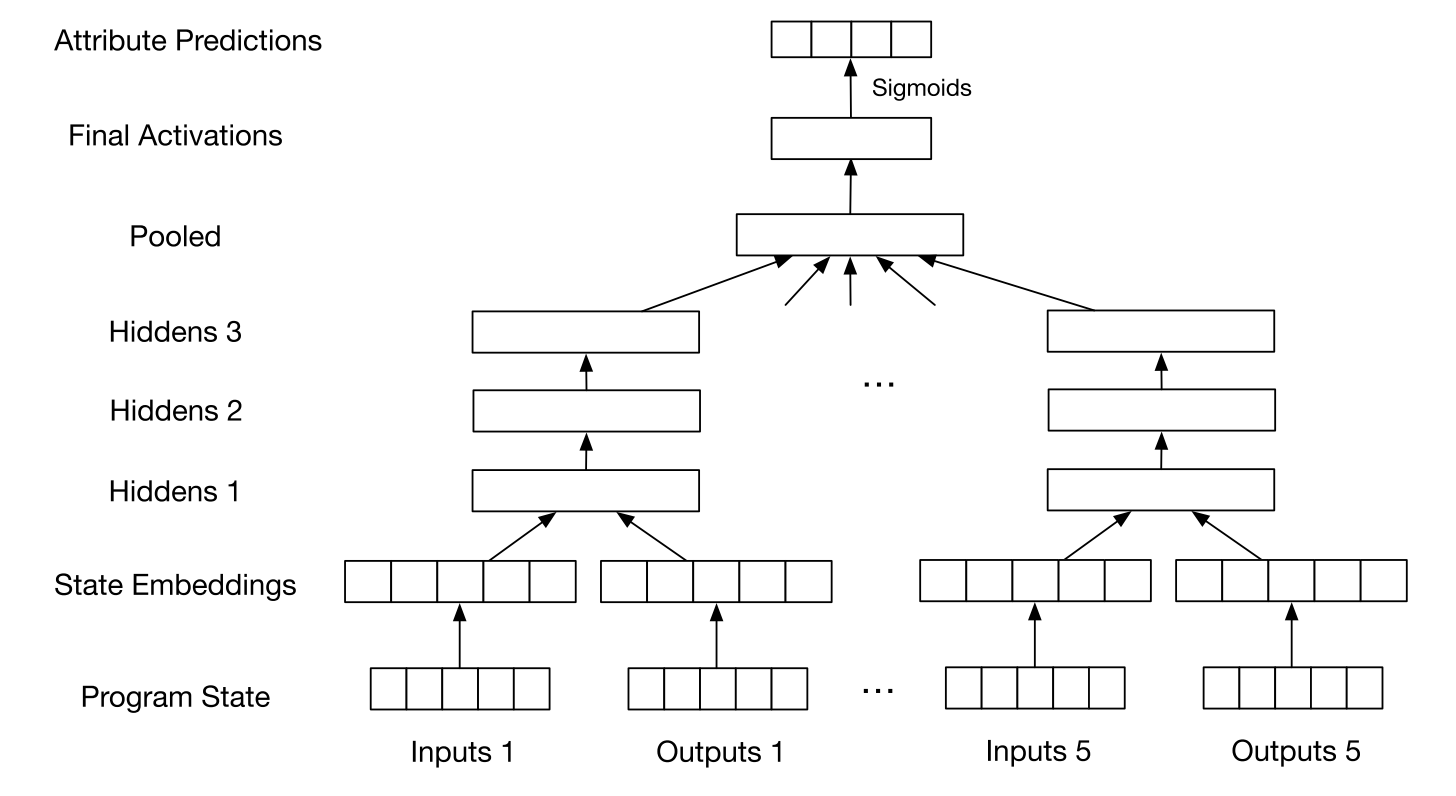The central idea in this paper is to use a neural network’s attribute predictions to condition the program search. There are a number of search strategies. The authors experiment with an optimized DFS, “sort and add” enumeration, Sketch, and $\lambda^2$, which can incorporate the attribution prediction as a prior to condition the search space. Many of these search algorithms are based on SMT solver approaches.

Remarkably, the authors report an order of magnitude speedup over baselines without the attribute predictions. DeepCoder is able to solve problems at the level of the easiest problems on programming competition databases: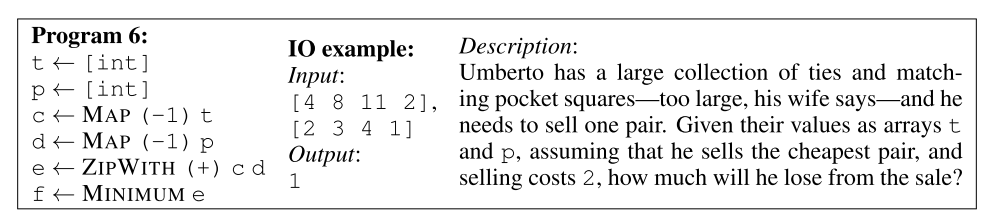Given the search space of programs of this size, this is a compelling research achievement.
DeepCoder combines both the symbolic and the connectionist paradigms in a pipeline that is able to learn interpretable programs. However, it remains to be seen whether input-output examples have sufficient information to permit useful attribute prediction for larger programs. Will this scale? We will probably need more attributes, as the authors note in their OpenReview thread.

## Differentiable Interpreters

How do we incorporate prior knowledge into a machine learning model? Bosnjak et al. 2017 consider this fundamental problem from a unique angle -- suppose the prior knowledge we have is procedural. They present an end-to-end approach to program learning, where a programmer can provide a program sketches with slots that need to be filled in. Gradient descent is then applied to “fill in” learned behavior from program input-output data.

The authors use the programming language Forth to conduct their work. They choose Forth in part because of its expressivity, but most importantly because its abstract machine is simple enough to implement a continuous approximation.

Forth’s abstract machine is represented by a state $S = (D, R, H, c)$, which contains two stacks. $D$ is a data evaluation pushdown stack, and $R$ is a return address pushdown stack. $H$ stores the heap, and $c$ is a program counter. A Forth program $P$ can be represented as a sequence of words $P = w_1 w_2 \dots w_n$, which can be regarded as a sequence of state transition functions. To make gradient based learning possible, the machine output must be differentiable with respect to these functions $w_i$. The author’s implementation, which they call $\delta 4$, models the Forth abstract machine as a recurrent neural network, parametrized by the transition functions at each time step.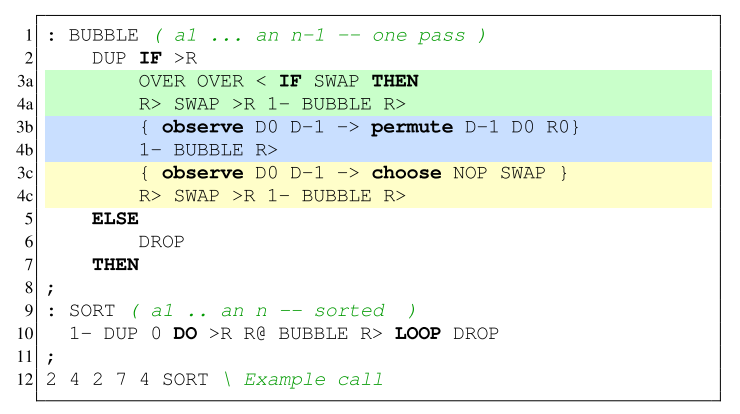In the above figure, there are two ways to provide a sketch for the sorting algorithm. In blue, the authors present the PERMUTE sketch, which specifies that the top two elements of the stack and the top of the return stack must be permuted based on the values of the former. In this setting, functionality to compare values and perform the permutation must be learned. In yellow, the authors write the COMPARE sketch, which provides additional procedural knowledge to the model. In this context, only the comparison between the top two elements in the stack must be learned.

Let $M$ represent a memory buffer corresponding to the data stack, return stack, or the heap. Then, differentiable read and write operations can be implemented as

$$\text{read}_\mathbf{M}(\mathbf{a}) = \mathbf{a}^T \mathbf{M}$$
$$\text{write}_\mathbf{M}(\mathbf{x}, \mathbf{a}) : \mathbf{M} \leftarrow \mathbf{M} - (\mathbf{a1}^T \odot \mathbf{M} + \mathbf{xa}^T)$$

Here, $\mathbf{a}$ represents an address pointer, and $\odot$ is elementwise multiplication. These operations are modeled after the way a Neural Turing Machine's memory functions (Graves et al. 2014).

A slot $w$ can be encoded by specifying a state encoder and a decoder. Users can choose from many operations for encoders and decoders, listed below.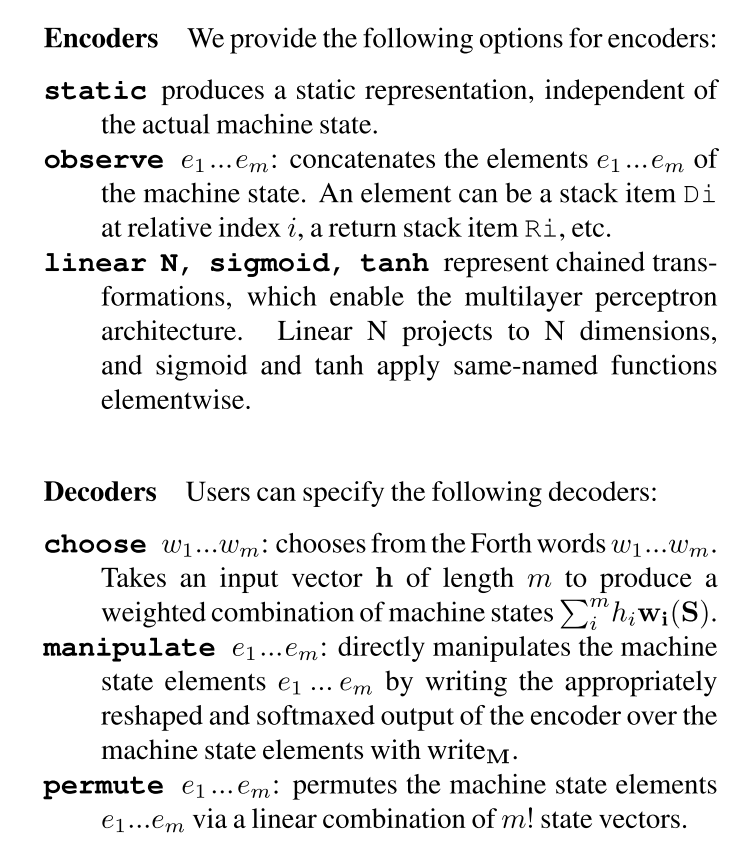Finally, a recurrent neural network is used to model execution. The RNN computes a state $S_{n+1}$ conditioned on a previous state $S_n$. We have:

$$\mathbf{S}_{n+1} = \text{RNN}(\mathbf{S}_{n}, \mathbf{P}_{\theta}) = \sum_{i=1}^{|P|} \mathbf{c}_{i} \mathbf{w}_{i} (\mathbf{S}_n).$$

This is quite computationally expensive. At every time step, a new machine state is calculated by executing all words in the program and then weighting the result states by the program counter. Thus, the authors use a number of optimizations to avoid full RNN steps. First, they use symbolic execution to “collapse” transition functions. For example, R > SWAP > R can be collapsed into a single transition. Second, they execute if branches in parallel and weigh their output states by the value of the condition.

This work is similar to probabilistic programming languages such as Church. In this setting, users can incorporate probabilities densities into programs to specify distributions over possible execution traces. While traditional probabilistic programming languages typically use a form of Bayesian inference to compute the posterior, $\delta 4$ updates parameters using gradient descent. The authors note that this style is similar to the TerpreT FMGD algorithm (Gaunt et al. 2016).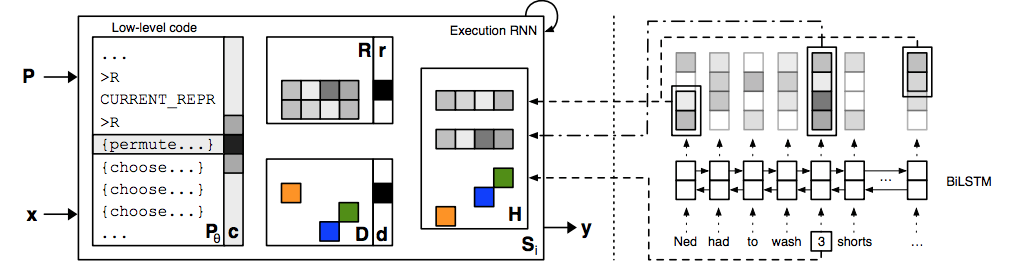The authors are able to learn a sorting procedure using only a few hundred training examples! Experimentally, they show that their D4 implementation vastly outperforms a Seq2Seq baseline. The seq2seq implementation fails to generalize at all to lengths longer than the training lengths. By contrast, the sketch highlighted earlier defines the outer loop which repeatedly calls the BUBBLE function; this guarantees that the functionality of the network is invariant to the input sequence length.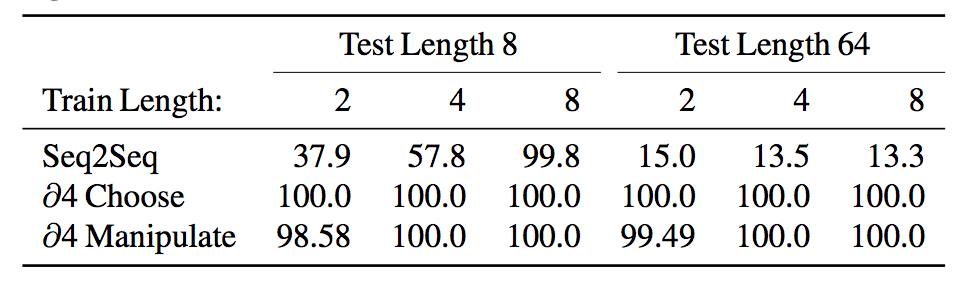## Unsupervised Learning By Program Synthesis

Given a dataset, how can we capture latent structure using a program? Ellis et al. 2017 applied program synthesis techniques uniquely to unsupervised learning. Examples include include visual concepts, linguistic rules, and multitask regression: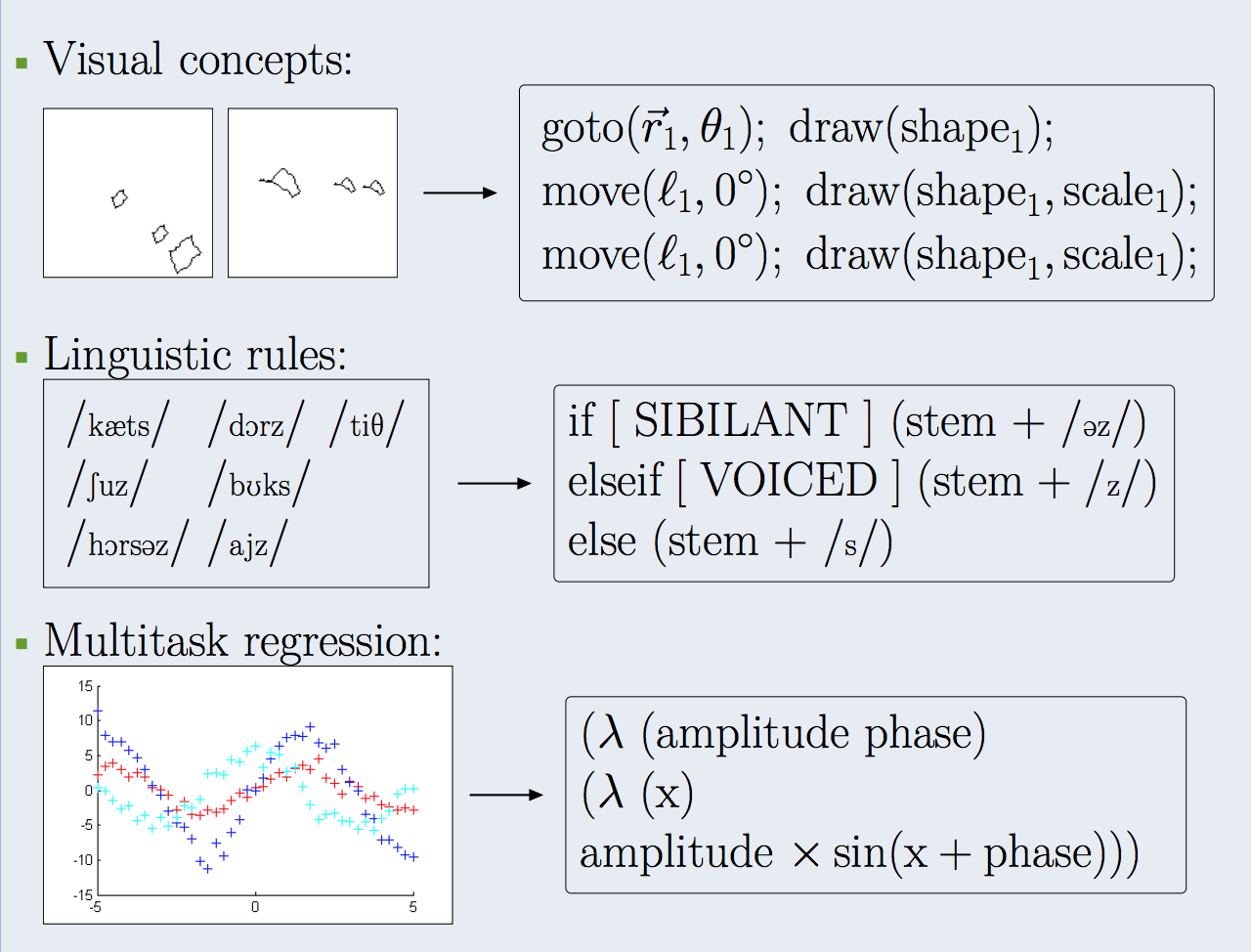More concretely stated, we would like to explain an observed dataset as the output of a program run on unknown inputs. We can formulate this joint inference over the program and the program inputs, optimizing for the smallest solution. The loss function is constructed as follows, with the objective of minimizing program length, data reconstruction error, and data encoding length.

$$\underbrace{- \log P_f(f)}_{\text{program length}} + \sum_{i=1}^{N} \left ( \underbrace{- \log P_{x|z} (x_i | f(I_i))}_{\text{data reconstruction error}} - \underbrace{\log P_I (I_i)}_{\text{data encoding length}} \right )$$

The authors again use the idea of a sketch, which they combine with symbolic search (namely, SMT solving), to produce a program.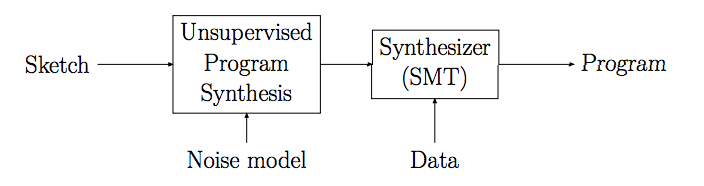The authors work with visual training examples from the Synthetic Visual Reasoning Test (SVRT), a test consisting of classification problems parsed into distinct shapes. They define a space of simple graphics programs referred to as “turtle programs” that can control the rendering of different shapes. The following context free grammar is used to construct “sentences” that can be mapped to different semantic models over visual concepts: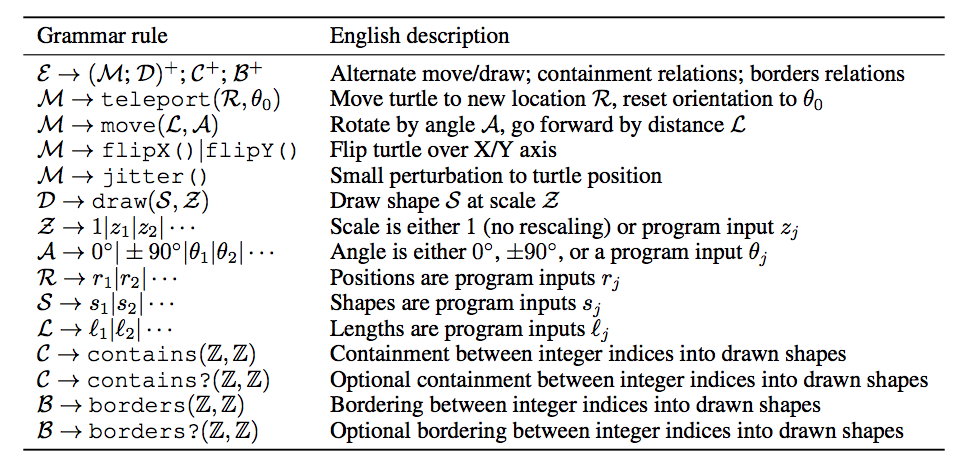A parser first analyzes each image and outputs structured information: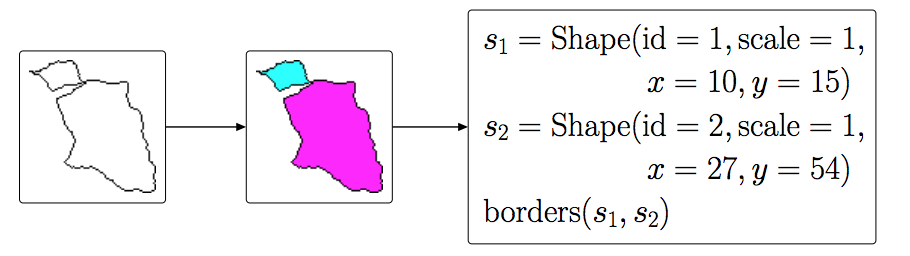Then their learning algorithm constructs a program from this parsed data: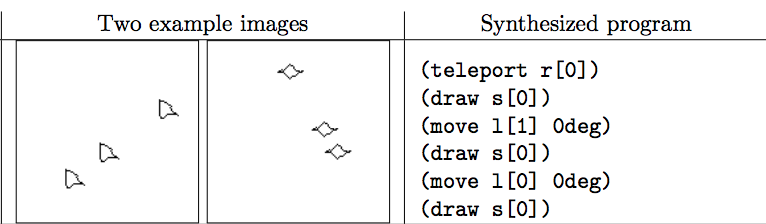The authors use 23 SVRT problems and evaluate the accuracy of their parser relative to several baselines. Unsupervised program synthesis outperforms a decision stump, convolutional network, and other discriminative baselines.